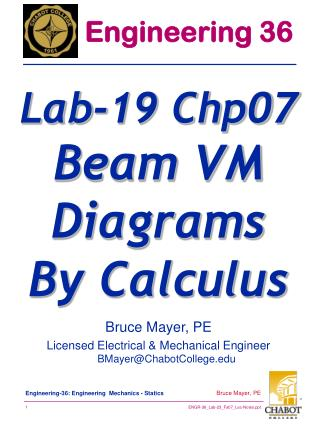Download PresentationBruce Mayer, PE Licensed Electrical & Mechanical Engineer BMayer@ChabotCollege.edu# Bruce Mayer, PE Licensed Electrical & Mechanical Engineer BMayer@ChabotCollege.edu - PowerPoint PPT Presentation

Download Presentation##### Bruce Mayer, PE Licensed Electrical & Mechanical Engineer BMayer@ChabotCollege.edu

Download Policy: Content on the Website is provided to you AS IS for your information and personal use and may not be sold / licensed / shared on other websites without getting consent from its author. While downloading, if for some reason you are not able to download a presentation, the publisher may have deleted the file from their server.

- - - - - - - - - - - - - - - - - - - - - - - - - - - E N D - - - - - - - - - - - - - - - - - - - - - - - - - - -
##### Presentation Transcript

1. Engineering 36 Lab-19 Chp07Beam VM DiagramsBy Calculus Bruce Mayer, PE Licensed Electrical & Mechanical EngineerBMayer@ChabotCollege.edu

2. Calculus Relations

3. MATLAB V&M Diagrams

4. MatLabCode - 1a % Bruce Mayer, PE * 31Jul07 % ENGR36 * problem 10.1.28 % file = Prob10_1_28_VM_Plot.m % % Find V & M functions by calculus % Plot Using this File %% % clear % clears memory % % Calculate PieceWise Shear-Force Functions x1 = 0:.1:4; v1= -12.5*x1.^2+246.67; x2 = 4:0.1:8; v2 = -100*x2+446.67; x3 = 8:0.1:10; v3 = -353.3*(x3./x3) x4 = 10:0.1:12; v4 = 300*(x4./x4); x5 = 12.01; v5 = 0; % % Combine the pieces by appending xv = [x1 x2 x3 x4 x5]; v = [v1 v2 v3 v4 v5]; % plot v(x); use formating tools to adjust appearance plot(xv,v) ,xlabel('x (ft)'),ylabel('V (lbs)'), title('P10.1.28 Vertically Loaded Beam'), grid display('Shear-force plot complete; hit any key to continue') pause %

5. MatLab Code – 1b % Calculate PieceWise Bending-Moment Functions m1 = -25*x1.^3/6 + 246.67*x1; m2 = -50*x2.^2 + 446.67*x2 - 266.67; m3 = -353.3*x3 + 2933; m4 = 300*x4-3600; % Combine the pieces by appending xm = [x1 x2 x3 x4]; m = [m1 m2 m3 m4]; % plot v(x); use formating tools to adjust appearance plot(xm,m) ,xlabel('x (ft)'),ylabel('M (ft-lbs)'), title('P10.1.28 Vertically Loaded Beam'), grid

6. MatLabCode - 2a % Bruce Mayer, PE * 30Nov10 % ENGR36 * S&T problem 10.1.28 % file = Prob10_1_28_VM_AREA_AnonFcn_1211.m % % Find V & M functions by calculus % Plot Using this File % % Set V Break-Points V0 = 246+2/3 V4 = V0 - 200 V8 = V0 - 600 V10 = 300 % % Define V functions V04x = @(x) -12.5*x.^2 + V0 V48x = @(x) -100*x + V0 + 200 V810x = @(x) 0*x + V8 V1012x = @(x) 0*x + V10 % % Set x, Calc V(x) x0m = -.001 V0m = 0 x04 = linspace(0,4); V04 = V04x(x04); x48 = linspace(4,8); V48 = V48x(x48); x810 = linspace(8,10); V810 = V810x(x810); x1012 = linspace(10,12); V1012 = V1012x(x1012); x12p = 12.001; V12p = 0; % % make solid line on x-Axis xax = linspace(-2,14); Vax = 0*xax; %

7. MatLabCode – 2b % concatenate x & V(x) vectors xAll = [x0m,x04,x48,x810,x1012,x12p]; Vall = [V0m, V04,V48, V810, V1012,V12p]; % % plot plot(xax, Vax, xAll,Vall, 'LineWidth', 3),xlabel('x (ft)'),ylabel('V (lbs)'),... title('P10.1.28 Vertically Loaded Beam'), grid display('Shear-force plot complete; hit any key to continue') pause % % Set M Break-Points M0 = 0; M4 = 720; M8 = 106 + 2/3; M10 = -600; % % Define M functions M04x = @(x) (-12.5/3)*x.^3 + V0*x; M48x = @(x) (-100/2)*x.^2 + (V0+200)*x - 200*(1+1/3); M810x = @(x) V8*(x-8) + M8; M1012x = @(x) V10*x - V10*10 + M10; % % calc M(x) using previous x vectors M0m = 0; M04 = M04x(x04); M48 = M48x(x48); M810 = M810x(x810); M1012 = M1012x(x1012); M12p = 0 % % concatenate M(x) vectors Mall = [M0m, M04,M48, M810, M1012, M12p]; %

8. MatLabCode – 2c % plot(xax, Vax, xAll,Mall, 'LineWidth', 3),xlabel('x (ft)'),ylabel('M (ft-lbs)'),... title('P10.1.28 Vertically Loaded Beam'), grid display('Bending-Moment plot complete; hit any key to continue') pause% % subplot(2,1,1) area(xAll,Vall),,ylabel('V (lbs)'),title('P10.1.28 Vertically Loaded Beam'), grid subplot(2,1,2) area(xAll,Mall),xlabel('x (ft)'),ylabel('M (ft-lbs)'),grid